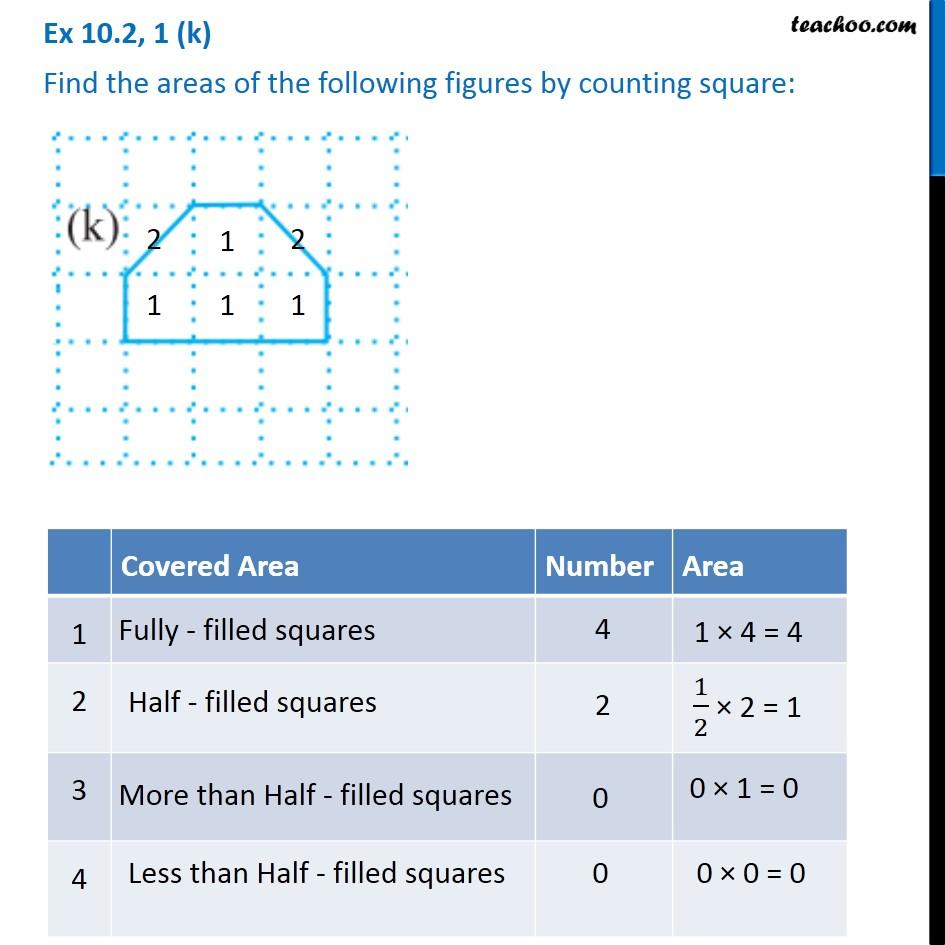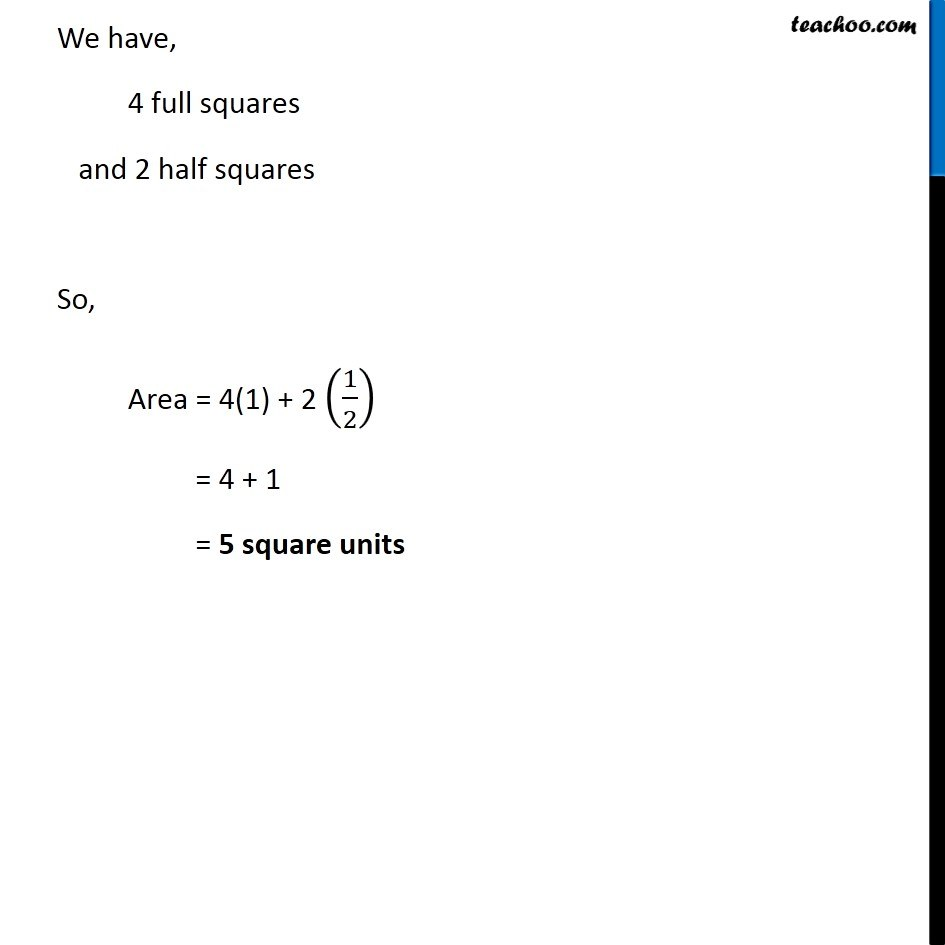Ex 10.2

Chapter 10 Class 6 Mensuration
Serial order wiseLearn in your speed, with individual attention - Teachoo Maths 1-on-1 Class

### Transcript

Ex 10.2, 1 (k) Find the areas of the following figures by counting square: Covered Area Number Area 1 Fully filled squares 4 1 × 4 = 4 2 Half filled squares 2 1/2 × 2 = 1 3 More than Half filled squares 0 0 × 1 = 0 4 Less than half filled squares 0 0 × 0 = 0 We have, 4 full squares and 2 half squares So, Area = 4(1) + 2 (1/2) = 4 + 1 = 5 square units